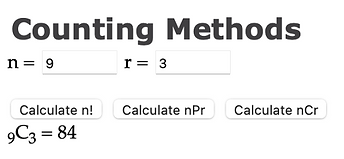top of page

## Chapter 5 - Day 9

##### Learning Targets
• Compute the number of combinations of n individuals taken r at a time.

• Use combinations to calculate probabilities.

• Use the multiplication counting principle and combinations to calculate probabilities.

Experience First

Wendy’s once claimed that there are 256 ways to personalize a Wendy’s hamburger. Today we will check the math.

Notice the stop sign at question #5 in the activity. Students should do well with questions #1-3 as they are a review of permutations from Lesson 5.7. Question #4 will be a challenge because we are introducing the idea that order of toppings doesn’t matter (mustard, ketchup tastes the same as ketchup, mustard!) You will need to debrief #1-4 before students can do the last two questions. Use question #4 to introduce the idea of combinations and be sure to make the connection to permutations (combinations are just a subset of permutations). We tell students to “divide out the repeats”.

In the end, we discover that Wendy must not have been paying attention in math class.

Formalize Later

Notice that the only formula we give for combinations is the one that relates it to permutations. We want students calculating a permutation first, then dividing by r! to eliminate the repeats.

Be ready for students to struggle a bit with the probability calculations in this lesson (especially with T. Swift). Getting practice is critical for student success here. The more combination probability questions they try, the better they get at these.

For calculating combinations, students can also use calculators or the “Counting Methods” applet at www.statsmedic.com/appletsbottom of page